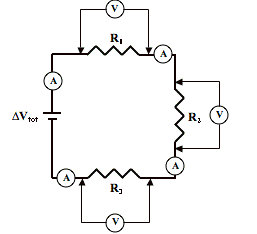# Electricity: Electric Ciruits

## Electric Circuits: Audio Guided Solution

#### Problem 25:Voltmeters can be used to determine the voltage across two points on a circuit. An ammeter can be used to determine the current at any given location on a circuit. The circuit at the right is powered by a 60.0-volt power source and utilizes three voltmeters and three ammeters to measure voltage drops and currents. The resistor values are 10.3 Ω (R1), 15.2 Ω (R2) and 12.8 Ω (R3). Determine the ammeter readings and voltmeter readings.

## Audio Guided Solution

Click to show or hide the answer!

## Habits of an Effective Problem Solver

• Read the problem carefully and develop a mental picture of the physical situation. If necessary, sketch a simple diagram of the physical situation to help you visualize it.
• Identify the known and unknown quantities and record them in an organized manner. Equate given values to the symbols used to represent the corresponding quantity - e.g., ΔV = 9.0 V; R = 0.025 Ω; I = ???.
• Use physics formulas and conceptual reasoning to plot a strategy for solving for the unknown quantity.
• Identify the appropriate formula(s) to use.
• Perform substitutions and algebraic manipulations in order to solve for the unknown quantity.